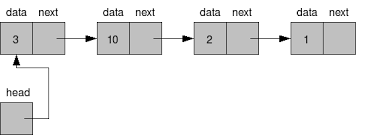Linked List is a very commonly used linear data structure which consists of group of nodes in a sequence.they are linear or sequential data structures in which elements are stored at non-contiguous memory location and are linked to each other using pointers.

Like arrays, linked lists are also linear data structures but in linked lists elements are not stored at contiguous memory locations. They can be stored anywhere in the memory but for sequential access, the nodes are linked to each other using pointers.

Each node holds its own data and the address of the next node hence forming a chain like structure.*Arrays can be used to store linear data of similar types, but arrays the size is fixed  on the other hand linked list are  dynamic in nature which allocates the memory when required.

*Insertion and deletion operations can be easily implemented where as in arrays its expensive as it needs shifting of elements

*Stacks and queues can be easily executed.

*Linked List reduces the access time.

*The memory is wasted as pointers require extra memory for storage.

*No element can be accessed randomly; it has to access each node sequentially.

*Reverse Traversing is difficult in link

*Not cache friendly. Since array elements are present at contiguous locations, there is a locality of reference which is not there in case of linked lists.

A linked list is represented by a pointer to the first node of the linked list. The first node is called the head node of the list. If the linked list is empty, then the value of the head node is NULL.

Each node in a list consists of at least two parts:1)data  2)Pointer (Or Reference) to the next node

In C/C++, we can represent a node using structure. Below is an example of a linked list node with integer data.

`struct Node{    int data;    struct Node* next; };`

In Java, LinkedList can be represented as a class and a Node as a separate class. The LinkedList class contains a reference of Node class type.

`class LinkedList {     Node head;  // head of list       /* Linked list Node*/    class Node     {         int data;         Node next;                    // Constructor to create a new node         // Next is by default initialized         // as null         Node(int d) {data = d;}     } }`

sample program in c/c++:

```#include<bits/stdc++.h>
using namespace std;

struct Node
{
int data; // Data
struct Node *next;  // Pointer
};

// Program to create a simple linked
// list with 3 nodes
int main()
{
struct Node* second = NULL;
struct Node* third = NULL;

// allocate 3 nodes in the heap in c++   |   // and in c
second = new Node;                       | second = malloc(sizeof(struct Node))
third = new Node;                        | third = malloc(sizeof(struct Node))

head->data = 1; //assign data in first node

// Link first node with the second node

// assign data to second node
second->data = 2;

// Link second node with the third node
second->next = third;

third->data = 3; //assign data to third node
third->next = NULL; //next pointer of the third block is made NULL to indicate that the linked list is terminated here

return 0;
}
```

let us now traverse through the created linked list:

keep moving the temp node to the next one and display its contents.
When temp is NULL, we know that we have reached the end of the linked list so we get out of the while loop.

in c++:    // in c we can use printf instead of cout
``` void printList(Node *node)
{
while (node != NULL)
{
cout<<node->data<<" ";
node = node->next;
}
}```

darkmode